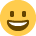# Financial Performance Exam

Hi all

Please help I have my Financial Performances Exam in two weeks and I can not get my head around the different variances :crying: I hav failed my mocks and really starting to stress, the green lights are not going well either, what can I do to help me?

Thanks

• Have a look at this thread

It relates to a budgeting question, but the last post by me explains how I calculate and remember some of the variances. I may not have explained it very clearly but have a read as that method certainly helped me.
• Thank you, I passed Bugeting just cant seem to get my head around this one.
• Is it calculating the variances, or explaining what they mean which is causing you problems?

I can't remember what is covered in each exam as I sat all the exams together so they kind of merged into one, but aren't the same variances tested in both Budgeting and Financial Performance?
• its trying to remember which one is which together with the Fixed O/heads ones
• Can someone please help me on this question in the osborne work book for practice paper 1 page.62. Does anyone know how to get the maximum labour cost and the target labour time per unit (minutes) ?
• Hello Salih

As before in your other requests for help, please can you post the question in full as not all students/members will have access to the Osborne work book.

Thanks.

JC
~ An investment in knowledge always pays the best interest ~
Benjamin Franklin
• Sure thing the question goes like this Omega Limited is considering designing a new product, and will use target costing to arrive at the target cost of the product. You have been given the following information and asked to calculate the target cost for labour so that the production manager can calculate the labour time that should be allowed for manufacture.

0) The price at which the product will be sold is £30.00
0) The company has firm orders for 20,000 units at the price for the first year
0) The fixed cost are £180,000 per year
0) The labour rate is currently £15 per hour
0) The required profit margin is 40%
0) The material requirement is 250 grams per unit
0) The material cost is £16 per kg.

a) Calculate the target time for the labour component of the product, using the following table,

sales price per unit is £30.00
Profit margin (£12.00) 30.00 X 405= £12.00
Total Cost £18.00
Fixed Cost £9.00 £180,000/20,000= 9.00
Material Cost £4.00 Not sure if this bit is right way i did it was 1000/250= 4 because 1000kg is 1 gram something like that
Maximum labour cost per unit(this bit i dont now how to get i done it by doing 18-9-4= 5 which is the correct answer but i know thats not the correct way to get the figure.

Target labour time per unit (minutes) answer is £20.00, but to get this would you times the material cost per unit by maumum labour cost per unit to get the target labour time?

This would be a wonderfull if you can help me out.

Thanks X:tongue_smilie:
• Your calculations all look right so far. You have also calculated the max labour cost per unit correctly (despite you thinking you haven't!)

So you know that there is £5 available for labour for each item. You are told that labour costs are £15 per hour, so you know that for each hour you need to produce at least 3 items (15/5), hence meaning you need to allow a max of 20mins for each item (60/3).
• hmm so everyting was fine expect the last bit, where you explained the max labour cost per unit part.
• Thank you so muchjust need to go over the written part :laugh:
• need help again im trying to use what you told me on this question here
0) price sold is £40
0) 10,000 units at a price of £40
0) fixed cost £120,000 per year
0) Labour requirement is 20minutes at a cost of £18.00 per hour
0) margin is 45%
0) Material is 200 grams per unit

Sales £40.00
Margin £18.00
Total cost £22.00
Fixed £12.00
Labour £6.00 (20X18/60= 6)
Maximum material cost per unit answer is 4 is it still the same way or is there anoher method to calculate this?

But for target cost per kilogram i dont know how to do it, would it be 10,000 unit divide by 200 grams then dvide it by 1000 from grams to kg giving you 20. 10,000/200= 50, 1000/50= £20.00
• This question is from aat elearning: A company purchases 3,500 kg of material at a cost of £72,000. The material price variance is £2,000 adverse. The standard price per kg is:

Dont know how to do this i thought i did gave my answer but it was wrong
• The question tells you that they paid £72,000 but this was £2,000 worse than they hoped to (or budgeted for). So you can work out the price that they hoped to buy 3,500kg for, and therefore the price they hoped to pay for 1kg (ie. the standard cost).

I think sometimes you need to forget the figures and re-phrase the question in your head. What are they telling you, and what are they asking you to find out. Re-phrase the question into every day English and it is less daunting.